This section is Section 2 of 3.

Speed as well as accuracy is important in this section. Work quickly, or you might not finish the paper. There are no  penalties for incorrect responses, only marks for correct answers, so you should attempt all 27 questions. Each question is worth one mark.

You must complete the answers within the time limit. Calculators are NOT permitted.

Good Luck!

Note – if press “End Exam” you can access full worked solutions for all past paper questions

Which of the following applies to both the nervous system and the endocrine (hormonal) system?

1.can be involved in homeostasis

2.can involve chemicals

3.can involve the brain

1.
0
0

## Explanation

All statements are correct. Both nervous and hormonal systems maintain a constant internal environment, hence they are homeostatic processes. Nervous systems use neurotransmitter chemicals in synapses, whereas hormonal systems use hormones as chemical messengers in the blood. Nervous responses are either voluntary (use the brain) or involuntary (do not use the brain), whereas hormonal responses are always involuntary, but are activated through detection in the brain.

Post Comment

The following chemicals are mixed together.

1. Pb(NO3)(aq) + Al(s)

2. 2KCl(aq) + 2F –(aq)

3. Al2(SO4)3(aq) + 3Fe(s)

4. CuSO4(aq) + Zn(s)

2. In which of the mixtures will a displacement reaction occur?
0
1

## Explanation

A displacement reaction will occur in 1 and 4 only. This is as, Aluminium is more reactive than Lead (Pb), and so will displace Ph from Pb(NO3). It is more reactive, as Al is in Group 3, whereas Pb is in Group 4. Hence, Al has less electrons in its outer shell, which can overcome nuclear attraction be lost easily, compared to lead which has more electrons, hence has to overcome a higher nuclear attraction. Zn (Zinc) is more reactive than Cu (Copper). This is as, for the same amount of shielding, (this is the number of electron shells, and as they are both in Period 4, they have the same number of shells – 4 shells) Zn has one more electron than Cu. The outermost electrons in both elements are in the 4s orbital. Zn has 2 electrons in the 4s orbital, whereas Cu only has 1 electron. Hence, Zn can easily lose these 2 electrons in the 4s orbitals for the same amount of shielding, compared to Cu.

Post CommentAnonymous Medicmind Tutor

Thu, 28 Oct 2021 18:40:38

Why is 2 not a displacement reaction as you surely displace the chloride ions?Anonymous Medicmind Tutor

Sun, 31 Oct 2021 18:07:47

thats what I thought toos Medicmind Tutor

Mon, 27 Jun 2022 07:08:52

i dont know why 2 is not a displacement reaction cause i am almost certain that fluorine will displace chlorine. Medicmind Tutor

Tue, 02 Aug 2022 12:24:43

Exactly, that's what I thought

Microwave, X-ray and infra-red radiation can all damage living tissues.

1. Microwaves cause damage because they are absorbed by water molecules.

2. X-rays cause damage because of their ionising ability.

3. Infra-red waves cause damage because of their ability to penetrate matter.

3. Which of the following statements correctly explain why this damage occurs?
0
0

## Explanation

Statements 1 and 2 are correct only. Microwaves can be absorbed by water molecules in your body, which can lead to damage. X rays cause ionisation, in which electrons off DNA molecules are released due to the energy of X-rays. This can cause DNA mutations, leading to cancerous cells. Statement 3 is incorrect, as infrared waves penetrate matter anyways, however this is not the reason for damage. The intensity of infrared waves can lead to damage (i.e. a very hot surface).

Post Comment

Given that x = 4.6 x 10⁷ and y = 2 x 10⁶, what is the value of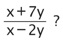4.
0
0

## Explanation

This question is a simple calculation. However, beware of your substitution and powers! First substitute any terms involving coefficients: y

7y = 7(2 x 106) = 14 x106 ; 2y = 2(2 x106) = 4 x106

(x+7y)/(x-2y) => x + 7y = 4.6 x 107+ 14 x106:convert all in terms of 106as this is the lowest power. Hence, 4.6 x 107= 46 x 106

Hence, (46 x 106) + (14 x 106) = 60 x106

x – 2y => (46 x106) – (4 x106) = 42 x106

Hence, 60 x 106/42 x 106= 10/7 (106cancels out completely)

Post Comment

1. Carbohydrase

2. Protease

3. Lipase

5. The action of which enzyme(s) could decrease the pH of a mixture of carbohydrate, protein and lipid?
0
0

## Explanation

The question asks for a decrease in pH, which corresponds to an increase in acidity of the mixture. Protease digests proteins into amino acids, lipase digests fats into glycerol and fatty acids, and carbohydrase digests starch into sugars. The action of protease and lipase will subsequently lead to an increase in acidity, as they produce acids – amino acids and fatty acids.

Sugars are of neutral pH, and so the action of carbohydrase will be ineffective in reducing pH.

Post Comment

The following reaction is at equilibrium:

2R(g) + 3S(g)  ↔ 2T(g)      ΔH is negative

which conditions (A–E) would produce most of the product T?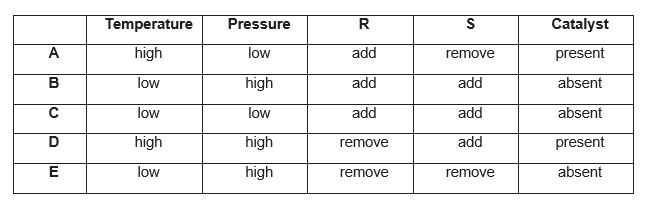6.
0
0

## Explanation

The question asks for the greatest product.

Temperature: The forward reaction is exothermic, due to negative enthalpy change. Hence, the forward reaction releases energy. Decreasing temperature favours the forward reaction, as more heat can be produced, hence the equilibrium shifts to the right, giving more product T. This is as, equilibrium shifts to oppose the decrease in temperature, by releasing more heat. Increasing the temperature would favour the reverse reaction, which is endothermic, as the heat can be absorbed to break bonds and produce more reactants.

Pressure: A high pressure favours the forward reaction, as the RHS contains fewer moles (2 moles), compared to the LHS (3 moles). The equilibrium shifts to oppose the increase in pressure, by giving product which has fewer moles. A decrease in pressure would favour the reverse reaction, as the LHS contains a higher moles than RHS.

R and S: Adding R would favour the forward reaction, as the equilibrium shifts to the right to oppose the increase in R, by producing more product. The same reason applies for S, as it is a reactant.

Catalyst: A catalyst has no effect on the attainment of equilibrium, but only affects (which is always to increase) the rate of attainment of equilibrium. Hence, the neither the presence nor absence of product T would affect the production of R. In this case, the option is ‘absent’, which is correct due to the above reason.

Post Comment

The diagram shows three appropriate meters, P, Q and R, connected in the conventional way in a The switch is initially open.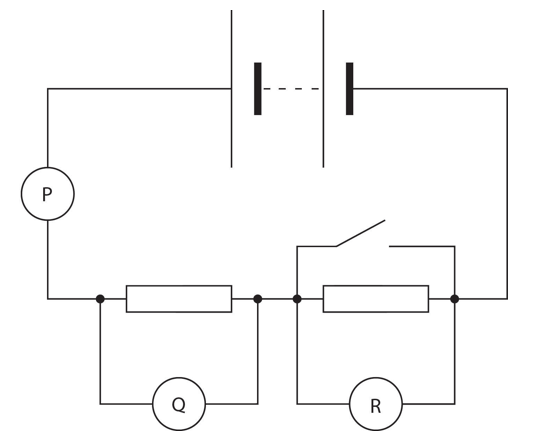7. The switch is now closed. What happens to the readings on each meter?
0
0

## Explanation

P, the ammeter, increases, as the current is not shared between the second resistor. Although the arrangement is series, and so current should remain constant in a series circuit, the short circuit causes the effects of a parallel circuit – current sharing – to be observed. The voltmeter Q increases, whereas the voltmeter across R decreases. This is as, short circuiting in R occurs, as the current bypasses the resistor, and flows through the path of the closed switch, as it has less resistance. The voltage is shared in that branch, compared to the branch with the resistor. Hence, the voltage drops in the path of the resistor, as measured by the voltmeter. Q increases, as no voltage is given to the resistor at R, hence more voltage is supplied to Q. Short circuiting: When a switch, in parallel to a component (in this case a resistor), is closed, the charge carriers prefer the path of least resistance, which in this case is the parallel wire with the switch. Hence, all charge flows through the wire and so none flows through the resistor. Consequently, the voltage measured is minimal. The reverse is true when the switch is open. The charge is forced to go through the resistor, and so the voltage is higher.

Post Comment

Simplify: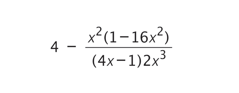8.
0
0

## Explanation

In order to simplify this expression, approach it in steps. First, recognise that (1-16) is a difference of 2 squares which can be split up into: (1-4x)(1+4x). Although the denominator is (4x-1), the minus sign can be applied to the (1-4x) bracket, giving (4x-1). Hence, the (4x-1) cancels out in both the numerator and denominator. The expression is now: 4 + ( (4x+1))/(2×3)

The and x³ can cancel out to obtain x in the denominator (in the rule of powers, x²-³ = x-1 = 1/x). The expression is now: 4 + (4x+1)/(2x) = 4 + 2 + 1/(2x) = 6 + 1/(2x)

((4x/2x) + (1/2x) = (4x+1)/(2x) = 4 + 2 + 1/(2x))

Post Comment

In a reflex action in which a person touches a hot plate and pulls their arm away, neurons of different lengths are Which answer identifies the relative lengths of the neurons?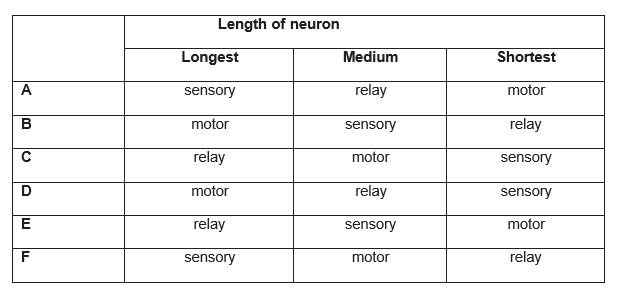9.
0
0

## Explanation

Sensory neurons have one long dendrite which receives impulses from receptor cells, and one short axon which takes the impulse to the CNS. This is the longest neurone. Relay neurons have many short dendrites which receive the impulses from sensory neurons, and many short axons which take the impulse to motor neurones. This is the shortest neurone. Motor neurons have many short dendrites which receive the impulses from the relay neurones in the CNS, and one long axon which takes the impulse to the effector muscle or gland.

Post Comment

1.15 g of sodium completely reacts with water at standard temperature and pressure (STP). What volume of hydrogen at STP is produced by this reaction?

[Assume in this question that 1 mole of any gas at STP has a volume of 22.4 dm³ (litres).]

[Ar values: H = 1, O = 16, Na = 23]

10.
1
0

## Explanation

The equation reaction is: 2Na + H2O Na2O + H2

Mole ratio of 2Na:H2 = 2:1

n(Na) = m/Mr = 1.15/23 = 0.05

Hence, H2 is 1/2 x 0.05 = 0.025

1 mole of any gas has 22.4dm³

Hence, 0.025 x 22400 cm³ = 560 cm³

Post Comment

The diagrams show two glass blocks in air. For the rays of light shown, the critical angle for the glass/air boundary is 42°. A ray of light is shown approaching the boundary in each case, with the angle of incidence labelled. Two possible labelled directions in which each ray might travel after reaching the boundary are also shown.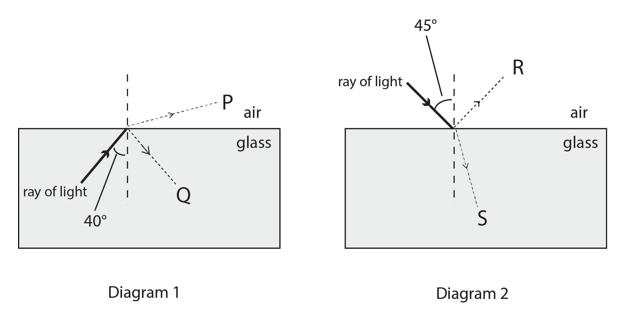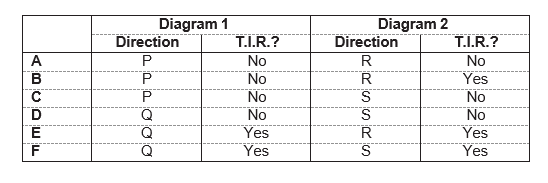11. Which line in the table correctly shows the direction taken by most or all of the light and also states whether total internal reflection (T.I.R.) will occur?
0
0

## Explanation

In Diagram 1, refraction occurs, as the light travels from a dense to a less dense medium. Most of the light is refracted in the direction of P, however, some internal reflection occurs. No T.I.R occurs, as the 40 < 42, and for T.I.R to occur, the angle of incidence must exceed the critical angle. In Diagram 2, the light follows the direction of S – refraction. This is as, the light travels from a more dense to a less dense medium. Although 45 > 42, the critical angle, the condition for T.I.R is that light travels from a more dense to a less dense medium. This is true for Diagram 1, yet the angle of incidence in that case doesn’t conform. In Diagram 2, the medium transfer is opposite.

Post Comment

The square ABCD is positioned so that its vertices are at the points with coordinates: (1, 1), (-1, 1), (-1, -1) and (1, -1).

The square is rotated clockwise through 90° about the origin and then reflected in the line y = x.

12. Which transformation will return the square to its original orientation?
0
0

## Explanation

Rotating the square clockwise through 90 degrees about the origin does not change the coordinates, as it is a square. Neither will the reflection in the line y=x affect the square; the coordinates will be interchanged, but the square will remain unmoved.

Beware, the question asks for the orientation of the shape. Hence, diagrams displaying coordinate movement will be useful.

Let A (-1,1), B (1,1), C (-1,-1), D(1,-1)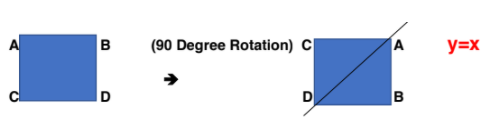(Reflection in y = x – points A & D = INVARIANT as they lie on the line. Only C and B are swapped diagonally)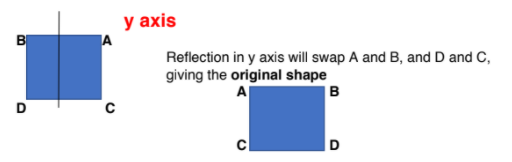Post Comment

Which of the following is not needed in order to genetically engineer bacterial cells to produce a fluorescent protein from a jellyfish?

13. Which of the following is not needed in order to genetically engineer bacterial cells to produce a fluorescent protein from a jellyfish?
0
0

## Explanation

The fluorescent protein is not required to genetically engineer bacterial cells to produce fluorescent protein from jellyfish. The gene for fluorescent protein production is required to be inserted in the plasmids of bacterial cells for fluorescent protein production. Genes code for a particular sequence of amino acids, which in turn codes for a particular protein. Options A,B and D are all required: a ligase enzyme seals and joins the sticky ends of the plasmid and inserted fluorescent protein gene, a plasmid carries bacterial DNA in which the fluorescent protein gene is inserted (the viral vector can transport the fluorescent protein gene to the bacterial DNA of bacterial cells – this mechanism is used by viruses to invade host cells). Restriction enzymes are needed to cut the fluorescent protein gene and the recipient plasmid. The recipient plasmid must have complementary sticky ends to the restriction site of the fluorescent protein gene, hence the same restriction enzymes are used.

Post Comment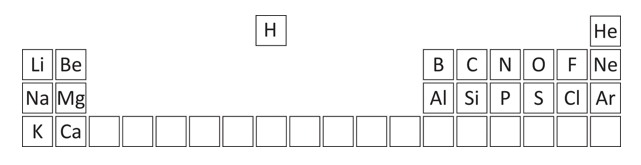14. Which one of the following pairs do not have the same electronic structure?
3
0

## Explanation

In order to simplify this question, you are given a part of the periodic table.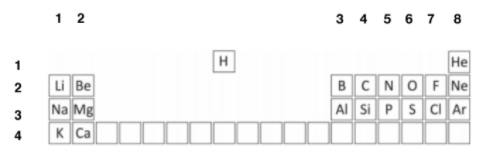Firstly, assign the groups and periodic numbers, in order to find out the electron numbers. The columns are the group numbers, and the rows are the periods.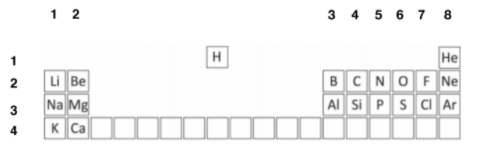The elements in question are: C, Mg, Cl, N, H, O, F, Ar and Ne. Carbon is in group 4, (4 outer electrons) and period 2 – hence, 2 shells. As maximum of 2 in innermost shell, C has: (2, 4) 2 + 4 = 6 electrons.

Mg: Mg is in Group 2, Period 3. Hence, 2, 8, 2 2 + 8 + 2 = 12 electrons

Cl: Group 7, Period 3. Hence, 2, 8, 7 2 + 8 + 7 = 17 electrons

F: Group 7, Period 2. Hence, 2, 7 2 + 7 = 9 electrons

N: Group 5, Period 2. Hence, 2, 5 2 + 5 = 7 electrons

H: Group 1, Period 1. Hence, 1 electron

O: Group 6, Period 2: 2, 6 2 + 6 = 8 electrons

Ar: Group 8, Period 3: 2, 8, 8 2 + 8 + 8 = 18 electrons

Ne: Group 8, Period 2: 2, 8 2 + 8 = 10 electrons

Post Comment

Two radioactive sources X and Y have half-lives of 4.8 hours and 8.0 hours respectively. Both decay directly to form only stable isotopes.

The activity of a sample of the source X was measured by a detector as 320 counts per minute, and simultaneously the radioactivity of a sample of the source Y was measured as 480 counts per minute. Immediately after the measurements, the two samples were combined.

[Assume that all readings in this question have been corrected for background radiation.]

15. What was the count rate when the activity of the combination of X and Y was measured 24 hours later?
0
1

## Explanation

24 hours is equal to 24/4.8=5 half lives for X and 24/8=3 half lives for Y. So the radioactivity of X would half 5 times in this period: 320/2=160,160/2=80, 80/2=40, 40/2=20, 20/2=10 counts per minute. The radioactivity of Y would half 3 times: 480/2=240, 240/2=120, 120/2=60 counts per minute. So the total activity of X and Y would be 10+60=70 counts per minute after 24 hours.

Post Comment

Three variables x, y and z are known to be related to each other in the following ways:

• x is directly proportional to the square of z
• y is inversely proportional to the cube of z.
16. Which of the following correctly describes the relationship between x and y?
0
0

## Explanation

We can express the proportionalities algebraically, by adding constants a (for expression 1) and b (for expression 2) to show equality: x = az²  ; y = b/()

Solve for k: a = x/() ; b = yz³

To equate the two formulae, the powers of z need to be the same. As  x = z5,raise the x expression by a power of 3, and the y expression by a power of 2.

z⁵= / , z⁵ = / Hence, / =  /

=   / ; Redefine a new constant of proportionality k =

= k/

Hence, the cube of x is inversely proportional to the square of y

Post Comment

Since Dolly the sheep, many other mammals have also been cloned by somatic cell nuclear transfer. The genetic material from a body cell is inserted into an egg cell that has had its own nucleus removed. The success rate ranges from 0.1% to 3%, which is why so few cloned animals have been produced.

1. An egg with a newly transferred nucleus may not begin to divide or develop.

2. The sperm cell may not fertilise the egg.

3. Implantation of the embryo into the surrogate mother might fail.

4. Implanted stem cells may not differentiate.

5.The enucleated egg and the transferred nucleus may not be compatible.

17. Which of the following are possible correct reasons why cloning may fail?
0
0

## Explanation

Statements 2 and 4 are incorrect. Statement 2 mentions fertilisation, whereas cloning does not involve this process; mitotic divisions occur to produce the new organism. Statement 4 mentions the implantation of stem cells; be careful with this, as it says ‘may not differentiate properly.’ Problems in differentiation may occur, however, the preceding use of ‘implanted stem cells’ is incorrect. Only the embryo is implanted into the womb of a surrogate mother, which contain stem cells. Stem cells alone cannot produce a live organism, as they are differentiated into specialised cells. Statements 1,3 and 5 are correct: there may be problems in division and development when the foreign nucleus is transferred to an enucleated egg – this issue of compatibility is stated in Statement 5 too. The implantation of the embryo into the surrogate mother can fail due to the rejection of the foreign embryo, as the foreign antigens on the surface of the embryonic cells can stimulate an immune response to destroy the embryo.

Post Comment

An impure sample of sodium hydroxide has a mass of 1.20 g. All the sodium hydroxide completely reacts with a minimum of 50.0 cm3 of 0.50 mol dm-3 hydrochloric acid.

[Ar values: H = 1; O = 16; Na = 23; Cl = 35.5]

18. What is the percentage purity of the sodium hydroxide sample?
1
3

## Explanation

To calculate percentage purity, we must compare the moles of NaOH and HCl.

n(HCl) = c x v x 10-3 = 50 x 0.5 x 10-3 = 0.025

The moles of NaOH and HCl are both the same as they completely react with each other.

Hence, n(NaOH) = m/Mr = 1.2/(23+1+16) = 0.03

Percentage purity: n(reacted)/n(NaOH) x 100 0.025/0.03 x 100 = 83.3%

Post Comment

Two resistors of R₁ ohms and R₂ ohms are connected in series to a battery which has an m.f. of V.

19. Which formula gives the power dissipated by resistor R₁?
1
2

## Explanation

P=IV and V=IR

P = I(IR) = I2R ; I = V/R ; V = emf V , R = R1 + R2

Hence, I = V/(R1 + R2)

P = (V/(R1 + R2))2 x (R1 + R2) = V2/ (R1 + R2)

Post Commentx Medicmind Tutor

Fri, 29 Oct 2021 22:17:00

why is the R1 there though ?x Medicmind Tutor

Fri, 29 Oct 2021 22:17:19

oh nvm i got it lmaoo

A solid shape is made by joining three cubes together with the largest cube on the bottom and the smallest on the top. Where the faces of two cubes join, the corners of the smaller cube are at the midpoints of the sides of the larger cube.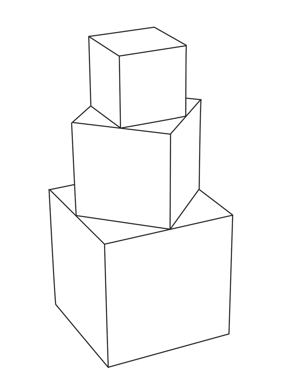20. The sides of the smallest cube have a length of 1 cm. What is the total surface area of the shape?
1
0

## Explanation

This is a very involved question, as it required the successive use of the

Pythagorean Theorem.

With the smallest cube, all sides are of length 1cm.

The vertexes of the cube are at the midpoint of the side of the second largest cube.

The length of the side of the cube, and 2 sides of the larger cube – which are bounded by 2 midpoints – form a triangle. There are 4 triangles on the second largest cube. As the lengths of the 2 sides bounded by the midpoints are equal, let each length = x. The hypotenuse of the triangle = 1.

+ =  2= 1  =1/2 Hence, x=1/√2

Area of 4 triangles: 4 (1/2 b x h) = 4 (1/2 x(1/√2)²) = 1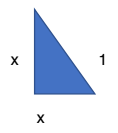The length of all sides of the second largest cube = 2(1/√2) = 2/√2

This becomes the length of the hypotenuse of the next triangle.

Using the above method,

+  = (2/√2)²2  2 =2  =1

Area of 4 triangles: 4 (1/2 b x h) = 4 (1/2 x  ) = 2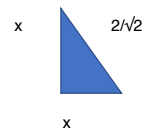The length of all sides of the largest cube = 2(1) = 2

The surface area of the entire shape:

Largest Cube: 5 sides – 5 x (area of each square) = 5 () = 20

4 triangles – 2

Second Largest Cube: 4 sides – 4 x (area of each square) = 4(2/√2)² = 8

4 triangles – 1

Smallest Cube: 5 sides – 5 x (area of each square) = 5 () = 5

Total Surface Area = 20 + 2 + 8 + 1 + 5 = 36cm²

Post Comment

1. gene for amylase

2. sex chromosomes

3. starch

21. Which of the following could be found in an adult liver cell?
1
0

## Explanation

An adult liver cell would contain sex chromosomes, as these determine the gender of the organism, and form the full set of chromosomes; hence, they are present in every cell (apart from sex cells which have either chromosome). Be careful – Statement 1 is correct – a gene for amylase is definitely present within an adult liver cell, as all genes are to be contained within a full set of chromosomes. However, the gene is not expressed in this cell, as amylase production is not required in the liver. Statement 3 is incorrect – starch granules are not present in liver cells, as they are too big to pass the cell membrane. Starch granules are present in chloroplasts of plant cells.

Post Comment

By using standard techniques to balance chemical equations and ensuring that the net charge is equal on both sides.

a C₂H₄O(aq) + Cr₂O₇²⁻(aq) + b H⁺(aq) → c C₂H₄O₂(aq) + d Cr³⁺(aq) + e H₂O(I)

22. Find the correct value for ‘ e ’ in the balanced equation above:
0
0

## Explanation

The half equation for Cr₂O₇²⁻ 2Cr³⁺: (Firstly, 7H₂O is added on the LHS, as there are 7O on the RHS initially. 14H⁺ added on the RHS, as to balance 14H on LHS (as 7H₂.

Then, Cr2 loses 6 electrons to form 2Cr³⁺ ; Cr₂O₇²⁻ 2Cr³⁺ + 6e-)

7H₂O + 2Cr³⁺ Cr₂O₇²⁻ + 14H⁺ + 6e-

Half Equation for C₂H4O C₂H4O₂: One oxygen atom is gained = oxidation = loss of electrons. 2 electrons are lost from the left hand side.

Hence, C₂H₄O C₂H₄O₂ + 2e-

Balance with water on the LHS: C₂H₄O + H₂O C₂H₄O₂ + 2e-

Balance with H+ on RHS: C₂H₄O + H₂O C₂H₄O₂ + 2e- + 2H⁺

Combining 2 half equations:

Equation 1: Cr₂O7²⁻ + 14H⁺ + 6e- 7H₂O + 2Cr³⁺

Equation 2: C₂H₄O + H₂O C₂H₄O₂ + 2e- + 2H⁺ (multiply this equation by 3 to cancel electrons as they do not appear in final equation):

Equation 3: 3C₂H₄O + 3H₂O 3C₂H₄O₂ + 6e- + 12H⁺

Combine Equation 1 and Equation 3: (6e- cancel out on both sides):

Cr₂O7²⁻ + 2H⁺ + 3C₂H₄O 3C₂H₄O₂ + 4H₂O + 2Cr³⁺

Hence, 4H₂O

Post Comment

Consider this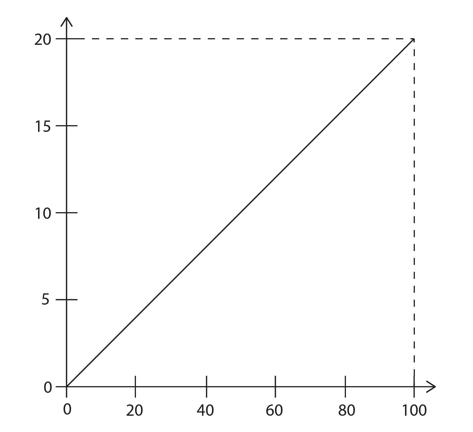23. Which one of the following could the graph not represent if all quantities are in S.I. units?
0
0

## Explanation

This is as, speed = wavelength x frequency wavelength = speed/frequency – if y =mx, then this graph would be y=m/x, hence it would give a reciprocal graph, which is not a linear graph, thus it would be incorrect.

Post Comment

A bag contains 8 blue and 2 red Three balls are chosen at the same time at random from the bag.

24. What is the probability that exactly two of the balls are the same colour?
0
0

## Explanation

Possible combinations of choices when exactly 2 balls of the same colour are chosen:

Choosing 2 blues and 1 red – BBR, BRB and RBB, which each have a probability of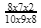=7/45. So collectively these 3 possibilities have a probability of 7/45×3=7/15.

Choosing 2 reds and 1 blue – RRB, RBR and RRB, which each have a probability of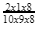=1/45. So collectively these 3 possibilities have a probability of 1/45×3=1/15.

So the overall probability would be 7/15+1/15=8/15.

Post Comment

Manx cats with two recessive alleles have a Heterozygous Manx cats lack a tail. Individuals with both dominant alleles die before birth.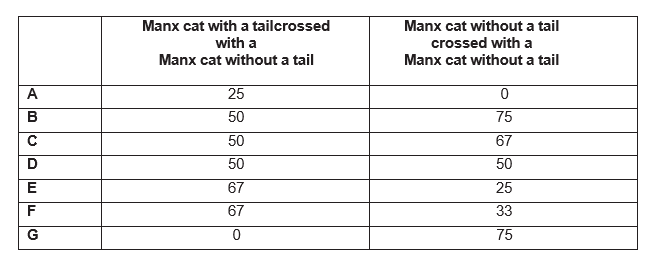25. Which answer shows the percentage of Manx cats without tails in a population for the two crosses given in the table?
0
0

## Explanation

Let’s define the recessive allele as t, and dominant allele as T. From the question: tt – tail; Tt –no tail; TT – die before birth. In order to find the population of Manx cats without tails, a genetic cross is needed. Any offspring with TT should be excluded in calculations, as they do not contribute to the outcome of a tail, due to pre-birth death.

With Tail X Without Tail

 t t T Tt Tt t tt tt

Without Tail X Without Tail

 T t T TT Tt t Tt tt

From the first genetic cross, there are 50% heterozygous dominant, hence without a tail, and 50% homozygous recessive – with a tail.

The second genetic cross requires essential care! The genotypes are: TT, Tt, Tt, tt. Here, the TT must be discarded. Hence, the sampling of heterozygous (no tail) has to occur from a population of the three genotypes: Tt, Tt and tt. This is 2/3, corresponding to 67% of the population.

Post Comment

The addition of NO as a catalyst to a mixture of SO₂ and O₂ speeds up the following reaction: SO₂ + ½O₂ → SO₃

The following reactions could be involved in the process.

1. N₂ + O₂ → 2NO

2. ½N₂ + O₂ → NO₂

3. NO + ½O₂ → NO₂

4. NO₂ → NO + ½O₂

5. SO₂ + NO → SO₃ + ½N₂

6. SO₂ + NO₂ → SO₃ + NO

26. Which one of the following shows the most likely course of the overall reaction?
0
0

## Explanation

These are the correct equations:

3.) NO + ½O₂ → NO₂

6.) SO₂ + NO₂ → SO₃ + NO

This is as, the NO is equation 3, in the reactants, cancels with the NO equation 6 – as one of the products. Also, NO₂ as a product of equation 2, cancels with NO₂ in reaction 6, in the reactants. This cancelling means that, NO and NO₂ are catalysts, as they do not partake in the overall equation: SO₂ + 1/2O₂ SO₃. NO is regenerated in equation 6, and NO₂ is used up in equation 6.

Post Comment

A resultant force of 20 N has accelerated a body of mass 4.0 kg from rest, until the present moment, at which time its kinetic energy is 1800 J.

27. If this force continues to act unchanged, how much extra kinetic energy will the body gain during the next 2 seconds?
0
1

## Explanation

F = ma for acceleration: a = F/m ; a = 20/4 = 5 ms-². Use 1⁄2mu² to find u = initial velocity: 1⁄2mu² = 1800

1⁄2 (4)(u²) = 1800 u² = 90 u = 30

V = u + at. (Where u (initial velocity) = 30). Hence, v = 30 + (2 x 5) = 40

KE = 1⁄2mv² = 1⁄2 x 4 x 402 = 3200

Hence, 3200 – 1800 = 1400

Post Comment

## Instructions

Below is a summary of your answers. You can review your questions in three (3) different ways.

The buttons in the lower right-hand corner correspond to these choices:

2. Review questions that are incomplete.
3. Review questions that are flagged for review. (Click the 'flag' icon to change the flag for review status.)

You may also click on a question number to link directly to its location in the exam.

## Instructions

This review section allows you to view the answers you made and see whether they were correct or not. Each question accessed from this screen has an 'Explain Answer' button in the top left hand side. By clicking on this you will obtain an explanation as to the correct answer.

At the bottom of this screen you can choose to 'Review All' answers, 'Review Incorrect' answers or 'Review Flagged' answers. Alternatively you can go to specific questions by opening up any of the sub-tests below.

x

## When should we call you?

It would be great to have a 15m chat to discuss a personalised plan and answer any questions

## What time works best for you? (UK Time)

Pick a time-slot that works best for you ?

Submit

Submit